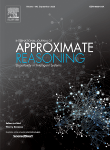### Archive

Archive for the ‘imprecise probability’ Category

## How to combine statistical methods

29 August 2012 1 comment

D. R. Bickel, “Game-theoretic probability combination with applications to resolving conflicts between statistical methods,” International Journal of Approximate Reasoning 53, 880-891 (2012). Full article | 2011 preprint | Slides | Simple explanationThis paper proposes both a novel solution to the problem of combining probability distributions and a framework for using the new method to combine the results of differing statistical methods that may legitimately be used to analyze the same data set. While the paper emphasizes theoretical development, it is motivated by the need to combine two conflicting estimators of the probability of differential gene expression.

## Confidence-based decision theory

D. R. Bickel, “Coherent frequentism: A decision theory based on confidence sets,” Communications in Statistics – Theory and Methods 41, 1478-1496 (2012). Full article (open access) | 2009 version | Simple explanation (link added 27 June 2018)To combine the self-consistency of Bayesian statistics with the objectivity of frequentist statistics, this paper formulates a framework of inference for developing novel statistical methods. The framework is based on a confidence posterior, a parameter probability distribution that does not require any prior distribution. While the Bayesian posterior is defined in terms of a conditional distribution given the observed data, the confidence posterior is instead defined such that the probability that the parameter value lies in any fixed subset of parameter space, given the observed data, is equal to the coverage rate of the corresponding confidence interval. Inferences based on the confidence posterior are reliable in the sense that the certainty level of a composite hypothesis is a weakly consistent estimate of the 0-1 indicator of hypothesis truth. At the same time, the confidence posterior is as non-contradictory as the Bayesian posterior since both satisfy the same coherence axioms. Using the theory of coherent upper and lower probabilities, the confidence posterior is generalized for situations in which no approximate or exact confidence set is available. Examples of hypothesis testing and estimation illustrate the range of applications of the proposed framework.

Additional summaries appear in the abstract and in Section 1.3 of the paper.

## How to use priors with caution

D. R. Bickel, “Controlling the degree of caution in statistical inference with the Bayesian and frequentist approaches as opposite extremes,” Electronic Journal of Statistics 6, 686-709 (2012). Full text (open access) | 2011 preprintThis paper reports a novel probability-interval framework for combining strengths of frequentist and Bayesian methods on the basis of game-theoretic first principles. It enables data analysis on the basis of the posterior distribution that is a blend between a set of plausible Bayesian posterior distributions and a parameter distribution that represents an alternative method of data analysis. This paper’s framework of statistical inference is intended to facilitate the development of new methods to bridge the gap between the frequentist and Bayesian approaches. Four concrete examples illustrate how such intermediate methods can leverage strengths of the two extreme approaches.